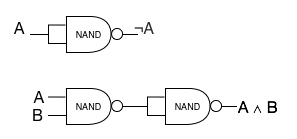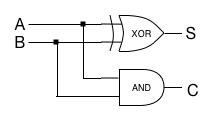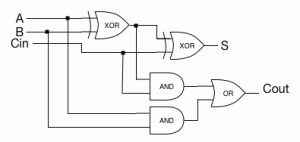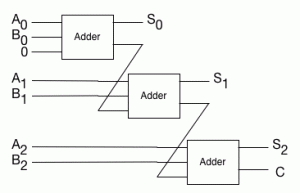# How Computers Really Work: Math via Boolean Logic

As I try to write more posts about ARM programming, I’m finding that I keep getting sidetracked by background stuff. Hopefully it’s interesting; if not, let me know in the comments!

Today’s sidetrack: how the heck does a computer actually do math?

As I said in my post about binary the other day, at a deep level, computers don’t actually work with numbers, not even zeros and ones. Computers are electronic devices, and what they really do is worth with electrical signals. In computer hardware, there are really just a few fundamental operations that it can perform with those signals. By taking those basic operations, and combining them in the right way, we can do math. How that works is very mysterious to most people. Obviously, I can’t describe all of how a computer works in a blog post. But what I’m going to do is explain the basic parts (the gates that are used to build the hardware), and how to combine them to implement a piece of hardware that does integer addition.

In computer hardware, there are four fundamental components that we can use to build operations, and they’re the basic operations of boolean logic: And, Or, Exclusive Or, and Not.

In talking about these kinds of operations, we frequently use truth tables to explain them. A truth table just lists all of the possible input combinations, and tells you what the output for them is. For example, here’s a truth table showing you AND, OR, and XOR:

A B A∧B A∨B A⊕B
0 0 0 0 0
0 1 0 1 1
1 0 0 1 1
1 1 1 1 0

In terms of hardware, you can actually implement all of these using one primitive gate type, the NAND (negated AND) gate. For example, in the diagram below, you can see how to build a NOT gate using a NAND gate, and then using using that NAND-based NOT gate, to build an AND gate using two NANDs.In the hardware, those gates are all that we have to work with. To build arithmetic, we need to figure out how to combine these primitive pieces to build up something that produces the right results. First, we need to be able to describe what the correct results are. We’ll start by figuring out what it means to add two 1-bit numbers. We’ll have two one-bit inputs. We need two outputs – because two one-bit numbers can add up to a two-bit sum. We’ll call the outputs S and C (sum and carry – S is the low-order bit, which would be the sum if we could only have a one-bit result, and C is the value of the second bit.)

We can describe addition with a truth table:

A B S C
0 0 0 0
0 1 1 0
1 0 1 0
1 1 0 1

We can read those rows as “If A is 0 and B is 0, then A+B is 0 with a carry of 0”.

If we compare that table to the table up above showing the basic operations, then we can see that S is exactly the same as XOR, and C is exactly the same as AND. So we can build a one-bit adder out of gates:This little one-bit adder is commonly called a half-adder, because to really implement a useful add operation, we need two of them for each bit.

If you think about what you’d need to do to add together two two-bit numbers, you couldn’t just use a half-adder for each bit. The carry from the first bit needs to get added to the second bit. You need to plumb the carry result from the first bit into a third input for the second bit. To get that second bit to work properly, you need to add together three one-bit values: the two inputs for the high-order bit, and the carry from the low-order bit. Generalizing, if you want to add together N bits,
when you’re computing the sum of the Mth bit, you need to include the carry from the M-1th bit.

To include the carry, we need to combine half-adders into a full adder, which looks like the following:Using that, we can create an N-bit adder, by chaining the carry output from bit M-1 to the carry input of bit M. This creates the simplest adder circuit, which is called a ripple-carry adder.Ripple-carry adders are the simplest way of building an integer addition operation in hardware. They’ve got one very big downside: to add the Nth bit, you need to have the result of adding the N-1th bit. That means that the more bits you add, the slower the ripple-carry adder gets. You have to way for the signals to propagate all the way through the circuit from the low bits to the high bits.

So ripple-carry isn’t really used in hardware anymore. There are a bunch of more complicated ways of building the adder that get rid of that propagation delay. It comes down to a very common tradeoff for engineers: performance versus complexity. But at the end of the day, the concept is still basically the same: it’s still a chain of multiple simple adders, that work the way I described here.

## 2 thoughts on “How Computers Really Work: Math via Boolean Logic”

1.Stuart

Typo alerts: “worth with electrical signals” (work with); “have to way for the signals” (wait).

Also, the other primitive gate type is the NOR gate: you can build all the other gates from that one gate. Just being thorough.